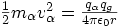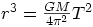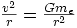## Stuck help

### AM4R

Posted 11 May 2007 - 05:05 PM

Im stuck on these questions. Can someone help?

1. An alpha particle with velocity of 2 x10^6 is fired at a target of gold foil in a vacuum. The mass of the alpha particle is 6.7 x 10^-27 and the atomic number for gold is 79.

Calculate the distance of closest approach.

2. r^3 = Gm/4pie^2 (T^2)

The orbital period of a geostationary satellite is equal to the period of rotation of the earth about its axis.

Calculate

the height of the satellite above the earths surface.
the speed of the satellite in its orbit.

Thanks

Posted 11 May 2007 - 05:34 PM

1) The distance of closest approach is the distance at which the electrostatic potential energy equals the kinetic energy the alpha particle had coming in, so:so rearranging this equation to make r the subject gives you an equation for the closest approach. You need to know the charge on an alpha particle and a gold nucleus, you're told that a gold nucleus has 79 protons in it, and you need to remember that an alpha particle has two protons in it. You need to know the mass of the alpha particle too, but that's given.

2) You're given. You actually know everything on the right hand side here, G is the gravitational constant, M is the mass of the earth (since we're thinking about a satellite orbitting the earth, and the mass of the satellite isn't given, it's a pretty good guess to go for M is the mass of earth). You are told that for a geostationary satellite, it has the same period as the earth about its axis, i.e. 24 hours = 86400s. So you can calculate r, which is the distance of the satellite from the centre of the earth (so you've got a little bit more to do if you want to find the height above the surface of earth).

For the next part, you need to equate the gravitational force and the central force for circular motion (since gravity is providing the central force when the satellite is in orbit), soCancel the mass of the satellite (which actually confirms the assumption we made about M being the mass of the earth, because the mass of the satellite cancels when you equate the forces) to getYou've just found r in the last part, so you can make v the subject and this will give you the speed of the satellite in orbit### AM4R

Posted 14 May 2007 - 09:38 AM

thanks ad